Beginner's tutorial, explains how to quickly look at your data or model predictions

## Viewing inputs and outputs¶

In this tutorial, we'll see how the same API allows you to get a look at the inputs and outputs of your model, whether in the vision, text or tabular application. We'll go over a lot of different tasks and each time, grab some data in a `DataBunch` with the data block API, see how to get a look at a few inputs with the `show_batch` method, train an appropriate `Learner` then use the `show_results` method to see what the outputs of our model actually look like.

## Vision¶

To quickly get access to all the vision functions inside fastai, we use the usual import statements.

```from fastai.vision import *
```

### A classification problem¶

Let's begin with our sample of the MNIST dataset.

```mnist = untar_data(URLs.MNIST_TINY)
tfms = get_transforms(do_flip=False)
```

It's set up with an imagenet structure so we use it to load our training and validation datasets, then label, transform, convert them into ImageDataBunch and finally, normalize them.

```data = (ImageList.from_folder(mnist)
.split_by_folder()
.label_from_folder()
.transform(tfms, size=32)
.databunch()
.normalize(imagenet_stats))
```

Once your data is properly set up in a `DataBunch`, we can call `data.show_batch()` to see what a sample of a batch looks like.

```data.show_batch()
```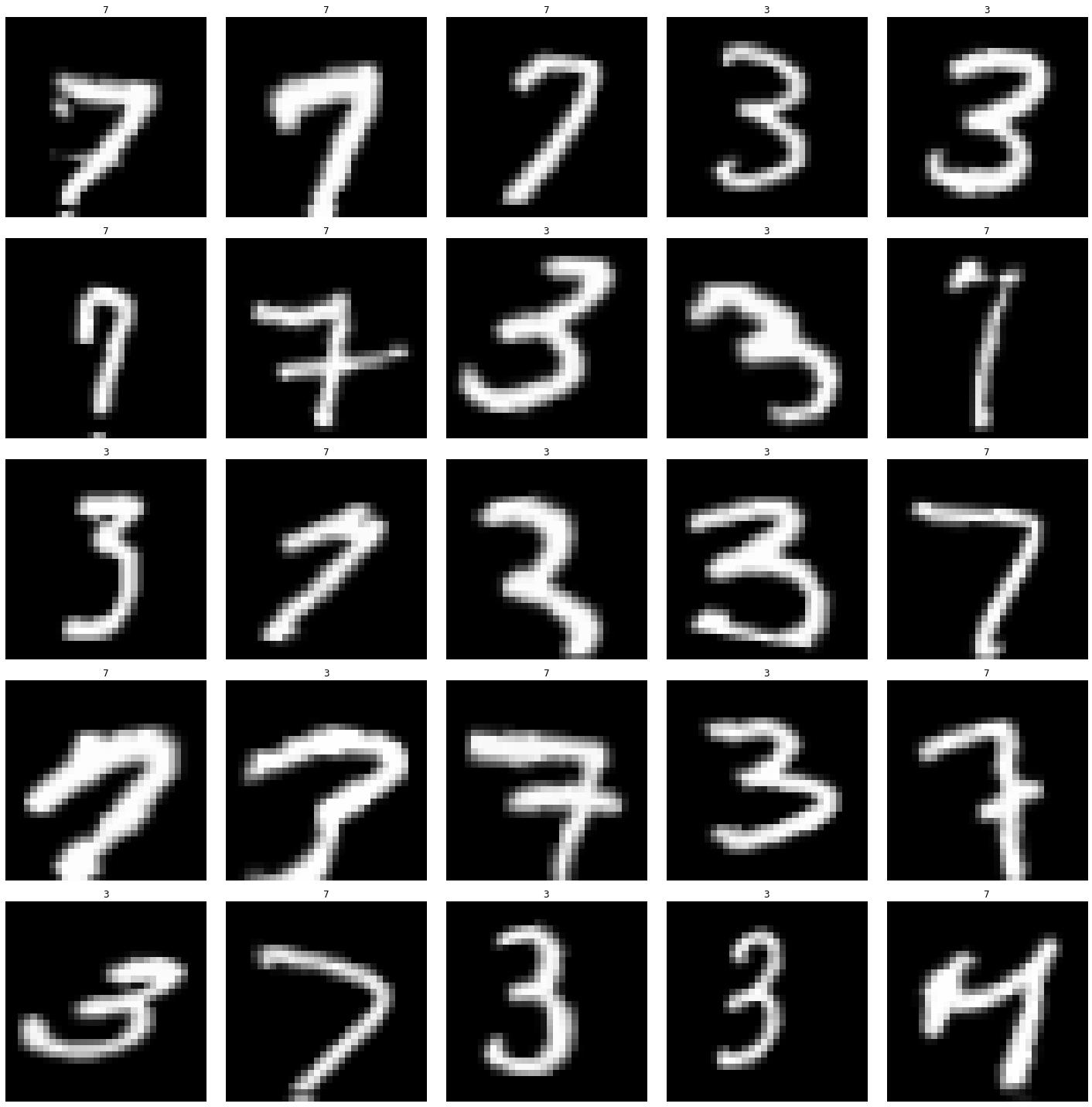Note that the images were automatically de-normalized before being showed with their labels (inferred from the names of the folder). We can specify a number of rows if the default of 5 is too big, and we can also limit the size of the figure.

```data.show_batch(rows=3, figsize=(4,4))
```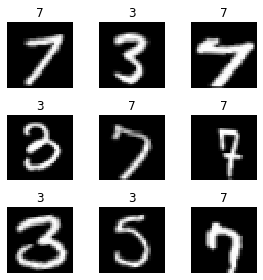Now let's create a `Learner` object to train a classifier.

```learn = cnn_learner(data, models.resnet18, metrics=accuracy)
learn.fit_one_cycle(1,1e-2)
learn.save('mini_train')
```
epoch train_loss valid_loss accuracy time
0 0.779994 0.744115 0.779685 00:01

Our model has quickly reached around 91% accuracy, now let's see its predictions on a sample of the validation set. For this, we use the `show_results` method.

```learn.show_results()
```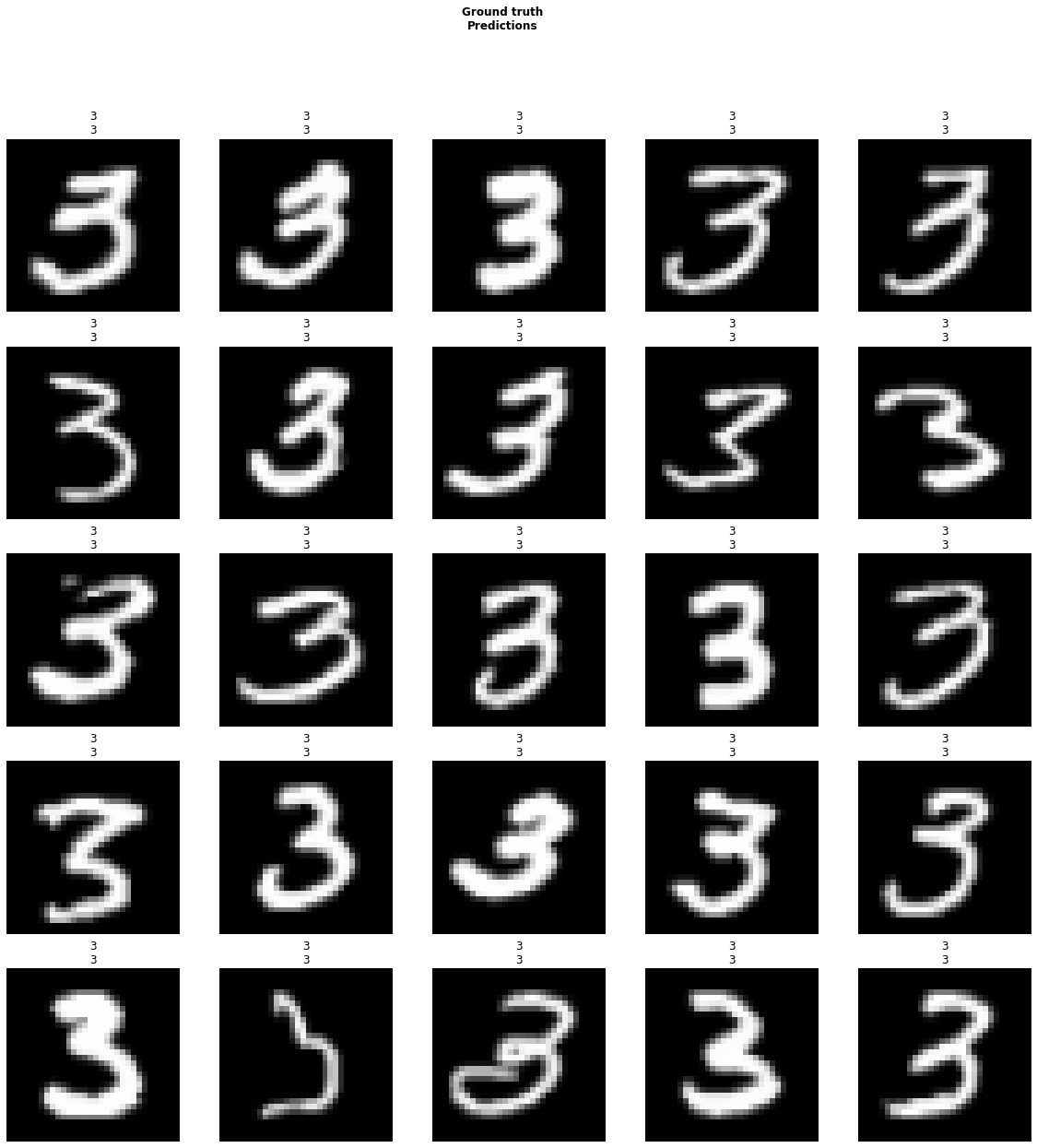Since the validation set is usually sorted, we get only images belonging to the same class. We can then again specify a number of rows, a figure size, but also the dataset on which we want to make predictions.

```learn.show_results(ds_type=DatasetType.Train, rows=4, figsize=(8,10))
```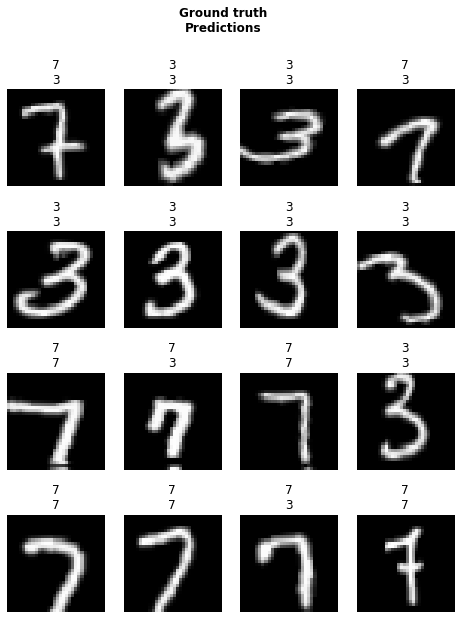### A multilabel problem¶

Now let's try these on the planet dataset, which is a little bit different in the sense that each image can have multiple tags (and not just one label).

```planet = untar_data(URLs.PLANET_TINY)
planet_tfms = get_transforms(flip_vert=True, max_lighting=0.1, max_zoom=1.05, max_warp=0.)
```

Here each images is labelled in a file named 'labels.csv'. We have to add 'train' as a prefix to the filenames, '.jpg' as a suffix and the labels are separated by spaces.

```data = (ImageList.from_csv(planet, 'labels.csv', folder='train', suffix='.jpg')
.split_by_rand_pct()
.label_from_df(label_delim=' ')
.transform(planet_tfms, size=128)
.databunch()
.normalize(imagenet_stats))
```

And we can have look at our data with `data.show_batch`.

```data.show_batch(rows=2, figsize=(9,7))
```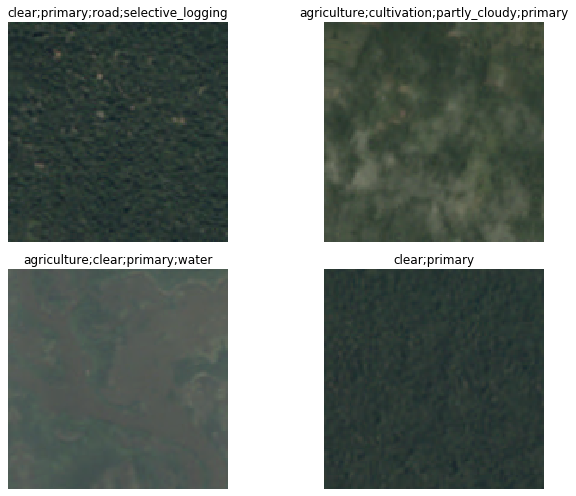Then we can then create a `Learner` object pretty easily and train it for a little bit.

```learn = cnn_learner(data, models.resnet18)
learn.fit_one_cycle(5,1e-2)
learn.save('mini_train')
```
epoch train_loss valid_loss time
0 1.024820 1.014537 00:01
1 0.948518 1.114616 00:01
2 0.887977 1.109744 00:01
3 0.839809 0.983482 00:01
4 0.794769 0.869911 00:01

And to see actual predictions, we just have to run `learn.show_results()`.

```learn.show_results(rows=3, figsize=(12,15))
```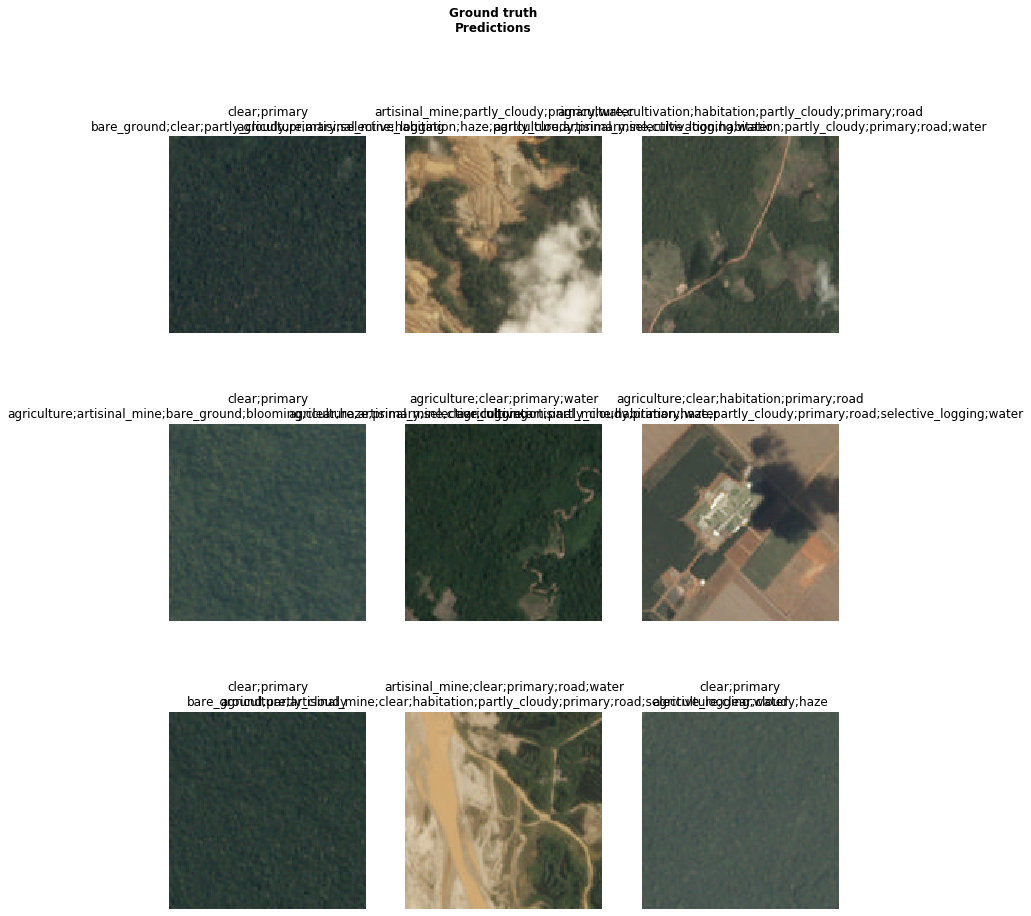### A regression example¶

For the next example, we are going to use the BIWI head pose dataset. On pictures of persons, we have to find the center of their face. For the fastai docs, we have built a small subsample of the dataset (200 images) and prepared a dictionary for the correspondance filename to center.

```biwi = untar_data(URLs.BIWI_SAMPLE)
```

To grab our data, we use this dictionary to label our items. We also use the `PointsItemList` class to have the targets be of type `ImagePoints` (which will make sure the data augmentation is properly applied to them). When calling `transform` we make sure to set `tfm_y=True`.

```data = (PointsItemList.from_folder(biwi)
.split_by_rand_pct(seed=42)
.label_from_func(lambda o:fn2ctr[o.name])
.transform(get_transforms(), tfm_y=True, size=(120,160))
.databunch()
.normalize(imagenet_stats))
```

Then we can have a first look at our data with `data.show_batch()`.

```data.show_batch(rows=3, figsize=(9,6))
```